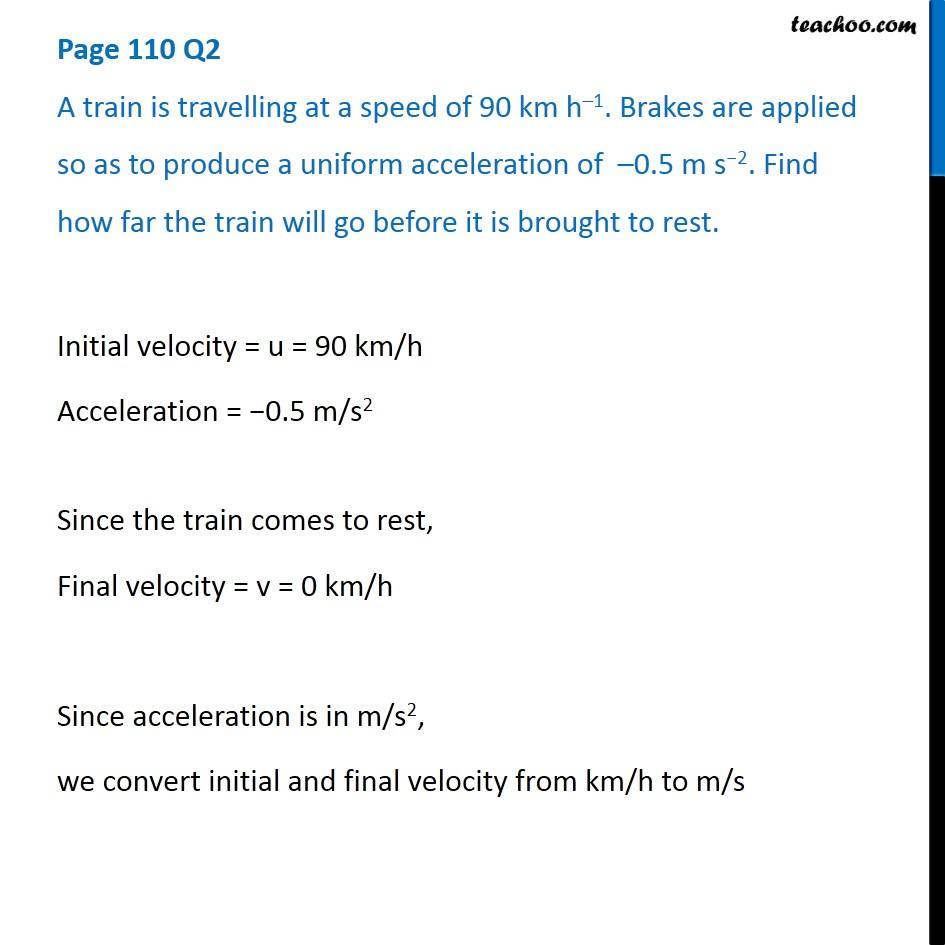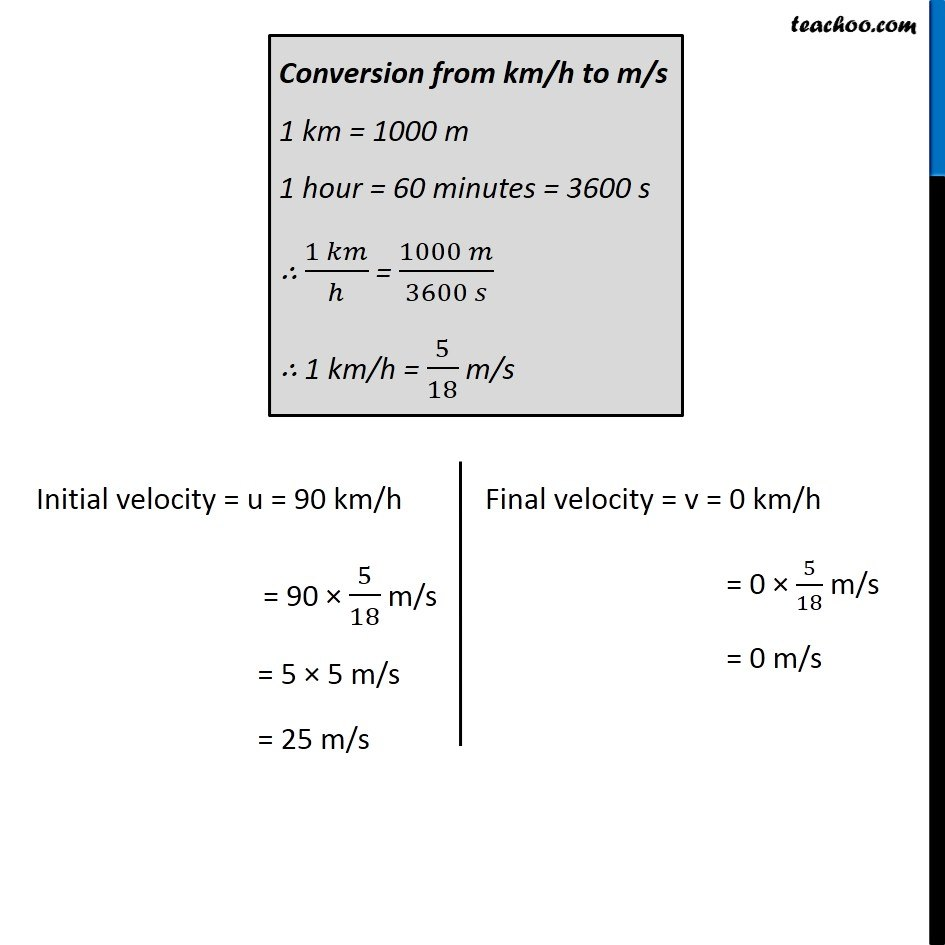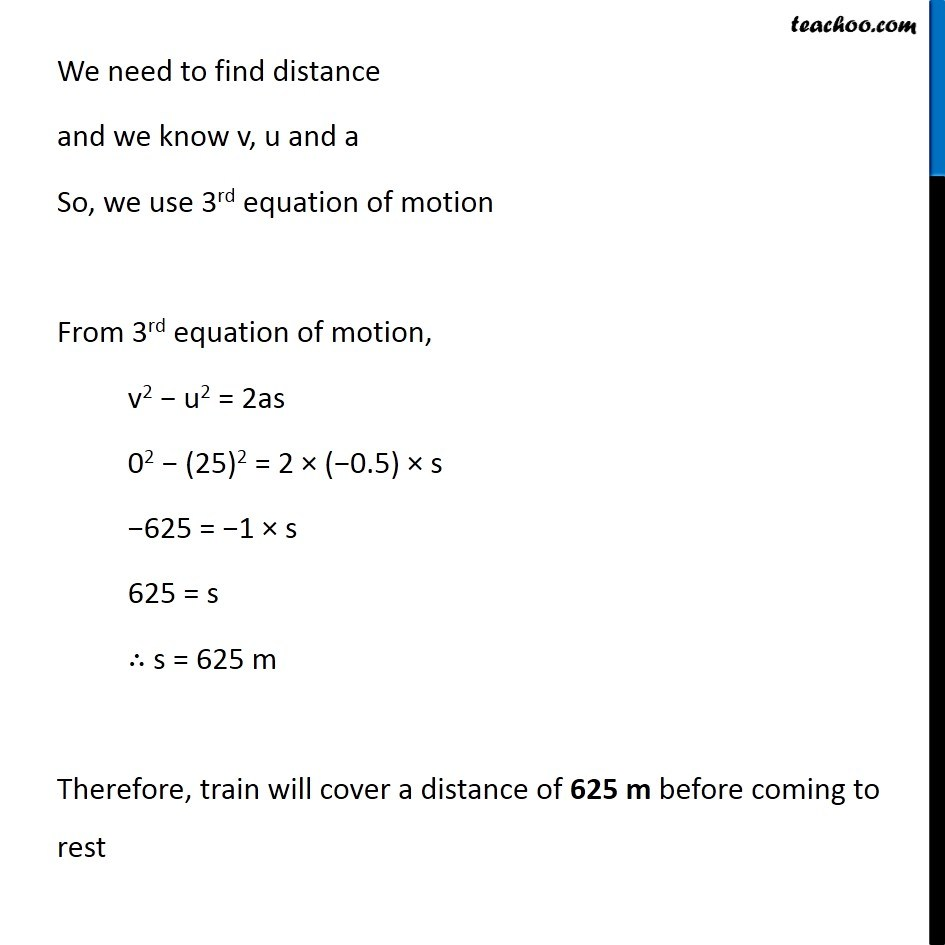Questions from Inside the chapter

Class 9
Chapter 8 Class 9 - MotionLearn in your speed, with individual attention - Teachoo Maths 1-on-1 Class

### Transcript

Page 110 Q2 A train is travelling at a speed of 90 km h–1. Brakes are applied so as to produce a uniform acceleration of –0.5 m s−2. Find how far the train will go before it is brought to rest. Initial velocity = u = 90 km/h Acceleration = −0.5 m/s2 Since the train comes to rest, Final velocity = v = 0 km/h Since acceleration is in m/s2, we convert initial and final velocity from km/h to m/s Conversion from km/h to m/s 1 km = 1000 m 1 hours = 60 minutes = 3600 s ∴ (1 𝑘𝑚)/ℎ = (1000 𝑚)/(3600 𝑠) ∴ 1 km/h = 5/18 m/s Thus, initial velocity = u = 90 km/h = 90 × 5/18 u = 25 m/s Final velocity = v = 0 km/h = 0 × 5/18 v = 0 m/s Conversion from km/h to m/s 1 km = 1000 m 1 hour = 60 minutes = 3600 s ∴ (1 𝑘𝑚)/ℎ = (1000 𝑚)/(3600 𝑠) ∴ 1 km/h = 5/18 m/s Initial velocity = u = 90 km/h = 90 × 5/18 m/s = 5 × 5 m/s = 25 m/s Final velocity = v = 0 km/h = 0 × 5/18 m/s = 0 m/s We need to find distance and we know v, u and a So, we use 3rd equation of motion From 3rd equation of motion, v2 − u2 = 2as 02 − (25)2 = 2 × (−0.5) × s −625 = −1 × s 625 = s ∴ s = 625 m Therefore, train will cover a distance of 625 m before coming to rest